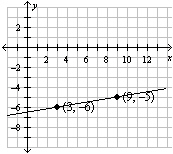Name:    Chapter B Test

Multiple Choice
Identify the choice that best completes the statement or answers the question.

Use the temperature scales to convert between Celsius and Kelvin.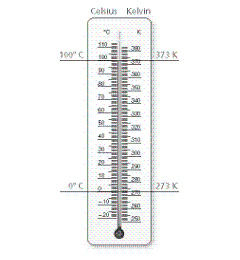1.

Water boils at 100°C. At what temperature does water boil on the Kelvin scale?
 a. 373 K c. 100 K b. 99 K d. –173 K

Solve the equation. Check your solution.

2.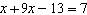a.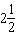c.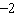b.d.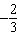3.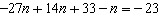a.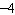c.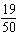b.d.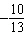4.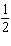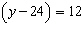a.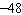c.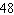b.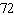d.Solve the equation. Check your solution.

5.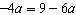a.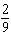c.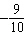b.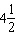d.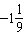6.

It costs \$120 to be a member of a music club. A member of the club pays \$5 per music lesson. A nonmember pay \$15 per music lesson. How many music lessons must a member and a nonmember take so the cost for each is the same?
 a. 6 c. 105 b. 12 d. 110

Use a table to solve the equation. Check your solution.

7.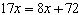a.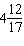c.b.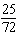d.8.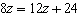a.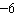c.b.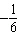d.Find x. Then find the perimeter of the square.

9.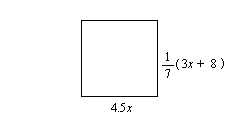a. x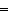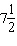; P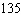units c. x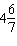; P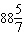units b. x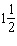; P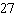units d. x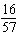; P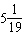units

Graph the line with the given slope that passes through the given point.

10.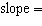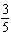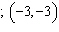a.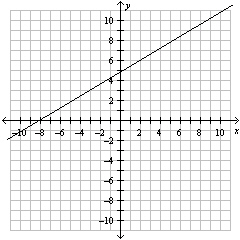c.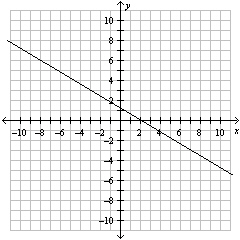b.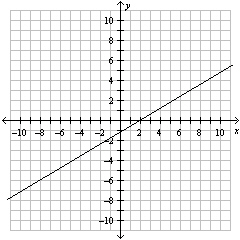d.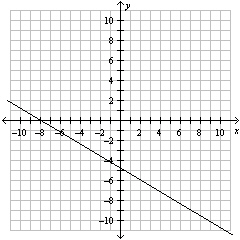Find the slope and y-intercept of the graph of the linear function.

11.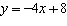a. slope: 8; y-intercept: –4 c. slope: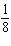; y-intercept: –4 b. slope: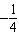; y-intercept: 8 d. slope: –4; y-intercept: 8

12.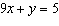a.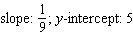c.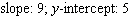b. slope: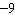; y-intercept: 5 d.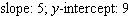13.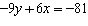a.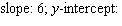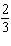c.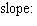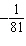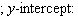b.; y-intercept: –81 d.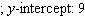Numeric Response

1.

Twenty-five more than 8 times the quantity of a number decreased by 9 is –23. Find the number.